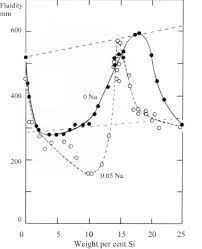## How to Calculate and Solve for Rate at Which Latent Heat is Evolved | Solidification of MetalsThe image above represents rate at which latent heat is evolved.

To compute for rate at which latent heat is evolved, three essential parameters are needed and these parameters are Density of Solidifying Metal (ρ’), Latent Heat of Fusion of Metal (Hf) and Mass Flow Rate (dM/dt).

The formula for calculating rate at which latent heat is evolved:

qx = ρ’Hf(dM/dt)

Where:

qx = Rate at Which Latent Heat is Evolved
ρ’ = Density of Solidifying Metal
Hf = Latent Heat of Fusion of Metal
dM/dt = Mass Flow Rate

Let’s solve an example;
Find the rate at which latent heat is evolved when the density of solidifying metal is 8, the latent heat of fusion of metal is 4 and the mass flow rate is 2.

This implies that;

ρ’ = Density of Solidifying Metal = 8
Hf = Latent Heat of Fusion of Metal = 4
dM/dt = Mass Flow Rate = 2

qx = ρ’Hf(dM/dt)
qx = (8)(4)(2)
qx = 64

Therefore, the rate at which latent heat is evolved is 64.

Calculating the Density of Solidifying Metal when the Rate at which Latent Heat is Evolved, the Latent Heat of Fusion of Metal and the Mass Flow Rate is Given.

ρ’ = qx / Hf (dM/dt)

Where;

ρ’ = Density of Solidifying Metal
qx = Rate at Which Latent Heat is Evolved
Hf = Latent Heat of Fusion of Metal
dM/dt = Mass Flow Rate

Let’s solve an example;
Find the density of solidifying metal when the rate at which latent heat is evolved is 25, the latent heat of fusion of metal is 5 and the mass flow rate is 3.

This implies that;

qx = Rate at Which Latent Heat is Evolved = 25
Hf = Latent Heat of Fusion of Metal = 5
dM/dt = Mass Flow Rate = 3

ρ’ = qx / Hf (dM/dt)
ρ’ = 25 / 5 (3)
ρ’ = 25 / 15
ρ’ = 1.67

Therefore, the density of solidifying metal is 1.67.
Continue reading How to Calculate and Solve for Rate at Which Latent Heat is Evolved | Solidification of Metals